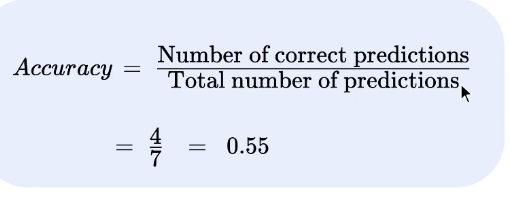Deep Learning — 6 Jars

Source: Deep Learning on MediumThe course is great to get started with deep learning and machine learning.

The professor devised a plan to set in order the process of learning DL and ML using six jars used to get in-depth with Deep Learning and understand how they fit in the field of AI.

But before stepping into learning about the actions of each jar lets briefly look at What is Deep Learning?

Deep learning is a subset of machine learning in artificial intelligence (AI) that has networks capable of learning unsupervised from data that is unstructured or unlabeled.

Jar 1: DATA

Data is the collection of facts in various forms such as numbers, words, etc. These data then has to be translated that a machine can process. In the ML concept, there can various kinds of problems that can be solved like classification, Regression problems.

The data then has to be translated into a language understandable by the machine and the given problem needs to be identified and the most suitable solution(algorithm) is selected to solve the problem.

The various kinds of problems and algorithms are regression, classification.

With the increasing amount of data, a task is training a machine to come out with output based on the given input.

Eg: Text Generation- it is a task achieved using deep learning models.

Jar 3: MODEL

The process of training an ML model involves providing a Learning algorithm with training data to learn from. For a given set of input data x and y, the True relation would be y = f(x).

These are some of the functions that belong to deep neural network, that we use to predict the model for the given input data.

Jar 4: LOSS FUNCTION

The above function is used to figure out the best function where n is the number of functions and the function having the least LF is considered as the best function.

varies loss function are:

any of the above loss function can be used to predict the best model.

Jar 5: LEARNING ALGORITHM

The best way to solve an algorithm is to try all the function that is a family of neural network function and to come out with the parameter value for which the loss function value is less.

These are some of the optimization solvers.

Jar 6: EVALUATION

Evaluation is the jar where the ML model is tested using the test data.

For example, considering the image classification model, the true model is tested with the ML model for accuracy.

The above is formula to check for Accuracy